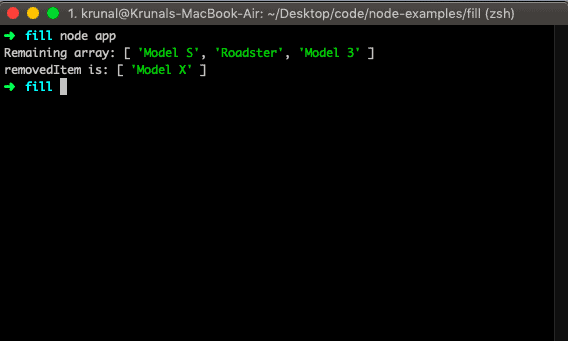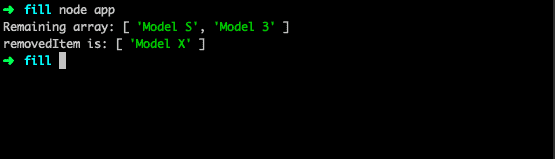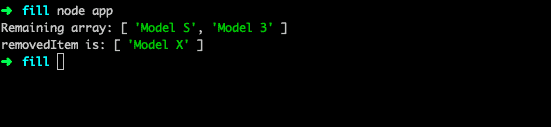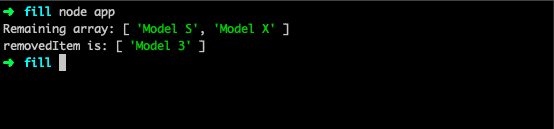# Javascript Array Splice: How to Splice Array in JavaScript

1
306Javascript array splice() is an inbuilt method that changes the items of an array by removing or replacing the existing items and/or adding new items. The splice() method adds/removes items to/from an array and returns the removed item(s). It will return a new array. In the splice method, if you specify the different number of the item to insert than the number you’re removing, then the array will have a different length at the end of the call.

## Javascript Array splice()

The splice method is similar to Array.prototype.slice, but unlike the slice() method it mutates the array it is called on. It also differs in that it can be used to add values to an array as well as remove them.

### Syntax

The syntax of the javascript array splice() method.

`array.splice(index, howmany, item1, ....., itemX)`

### Parameters

So here the first parameter is the index of an array which means where to start the adding or to remove the item. The second parameter is howmany means from the start index how many indexes we need to cover from the starting index.

Then the last parameters are optional. If we specify, then it will add the items on the array at specifying the starting index.

Let us take a simple example by creating a file called app.js and add the code.

```// app.js

let tesla = ['Model S', 'Model X', 'Model 3'];
let removedItem = tesla.splice(1, 1, 'Roadster');

console.log('Remaining array:', tesla);
console.log('removedItem is:', removedItem);```

So, here we have defined one array, and then we are changing that array by providing the first argument as a starting point of an index which is 1. So it will look for index 1 which is Model X. Now the second parameter is also 1.

So it will be a length of how many indexes we need to go to the right side of an array. So in our case, it is still Model X. So the third parameter Roadster will replace the Model X.Now, if we do not specify the third or fourth argument, then it will remove the item from an array. It will take as removing an item from the array.

If you are a Javascript developer then you must have to use the splice() method to remove the data based on ID. We do lots of these stuff on a framework like Angular, React, or Vue.js.

```// app.js

let tesla = ['Model S', 'Model X', 'Model 3'];
let removedItem = tesla.splice(1, 1);

console.log('Remaining array:', tesla);
console.log('removedItem is:', removedItem);```

That means the Model X will be removed from an array and we get the remaining array.## If we do not specify the second argument

Let us see the scenario, where we do not determine the second argument as well.

```// app.js

let tesla = ['Model S', 'Model X', 'Model 3'];
let removedItem = tesla.splice(1);

console.log('Remaining array:', tesla);
console.log('removedItem is:', removedItem);```

If we do not specify the second argument, then it will take the first argument and find that index and remove that item and other items from that index. So in the above example, we have given the index 1 which means it will go to index 1 and start removing all the items from that index and give the remaining item. In our case, it is the Model S.## If the starting index is negative(-)

Now, let us take a scenario, where the starting index of an array is negative. That means it will count backward at the end of an array.

```// app.js

let tesla = ['Model S', 'Model X', 'Model 3'];
let removedItem = tesla.splice(-1);

console.log(tesla);
console.log(removedItem);```

In the above example, it will start backward and remove the first item from the backward point of view. In our case, it is Model 3. So it will remove that, and if we check the Tesla array, then we only got two items in the array now.Finally, Javascript Array splice() example is over.

Javascript array indexOf()

Javascript array lastIndexOf()

Javascript array findIndex()

Javascript array reverse()

Javascript array isArray()

#### 1 Comment

1.Amit Parmar

thanks for the post

This site uses Akismet to reduce spam. Learn how your comment data is processed.